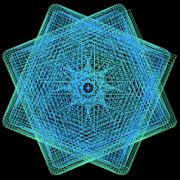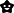GeometricsEveryoneFamily Friendly
571
- With Geometrics, you are the creator, discoverer, artist, and mathematician.
- Advanced users can set the equation properties manually.
- Images can be saved to your device.

TRIGONOMETRY OVERVIEW:

A polar graph represents a math equation where the distance [r] from the center of the graph is determined by an equation. [r=1 is a circle. r=sin(angle) makes loops]
Polar Graph:
http://en.wikipedia.org/wiki/Polar_graph

Normally you start the angle from 0 and go all the way around to 360 degrees incrementing by some small amount. When you skip faster around the circle in a regular interval (say every 80 degrees), you get something interesting. A Maurer Rose http://en.wikipedia.org/wiki/Maurer_rose

Geometrics takes this maurer rose idea and puts you in control of the design:
The amount to skip, and a multiplier for the angle (to determine how many petals your maurer rose will have).

DESCRIPTION OF CONTROLS:

1. Swipe left or right - Restart the pattern changing the number of "flower petals"

2. Swiping up and down - Restart the pattern changing the "amount of skip"

3. Pressing and holding - Cycle through 3 trigonometric math functions (single sin equation, multiple sin equations, and a tan equation)
Collapse

Review Policy
2.5
571 total
5
4
3
2
1

What's New

* Fixed the ability to save the spiral graph image to external storage.
Collapse

Updated
October 2, 2017
Size
1.9M
Installs
100,000+
Current Version
11
Requires Android
4.0 and up
Content Rating
Everyone
Permissions
Offered By
Kyle Fischer
Developer
817 Nordyke Road Cincinnati, Ohio 45255